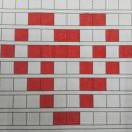墨滴2021/11/13  阅读：106  主题：默认主题

# 一文详解力扣int *returnSize和int** returnColumnSizes

### 三数之和

#### 示例：

``输入：nums = [-1,0,1,2,-1,-4]输出：[[-1,-1,2],[-1,0,1]]    输入：nums = []输出：[]    输入：nums = 输出：[]``

#### 提示：

• `0 <= nums.length <= 3000`
• `-105 <= nums[i] <= 105`

#### 默认的代码模板

``/** * Return an array of arrays of size *returnSize. * The sizes of the arrays are returned as *returnColumnSizes array. * Note: Both returned array and *columnSizes array must be malloced, assume caller calls free(). */int** threeSum(int* nums, int numsSize, int* returnSize, int** returnColumnSizes){}``

### 分析

• 返回大小为 `*returnSize` 的数组数组。
• 数组的大小作为 `*returnColumnSizes` 数组返回。
• 注意：返回的数组和 `*columnSizes` 数组都必须被 `malloced`，假设调用者调用 `free()`

``int main(){    int nums = {-1, 0, 1, 2, -1, -4};    int numsSize = 6;    int returnSize;// 表示返回的二维数组的行数    int **returnColumnSize;// 指向列数组指针的指针    // 注意：列数组在哪我们无从得知，也不需要知道，    // 我们只要知道有个一阶指针指向它就行了，我把它叫做列数组指针    int **ans = threeSum(nums, numsSize, &returnSize, returnColumnSizes);    // &returnSize 引用传值    // 最后一个参数我认为也可以是 int *returnColumnSize，然后传入&returnColumnSizes，效果是一样的    for (int i = 0; i < (*returnSize); i++) {        for (int j = 0; j < (*returnColumnSize)[i]; j++)            printf("%d", ans[i][j]);        printf("\n");    }    return 0;}``

``int **threeSum(int *nums, int numsSize, int *returnSize, int **returnColumnSizes){    // ans是二维数组，先假设有100000个答案，准备有100000个一阶指针的空间先    // 每个一阶指针可以指向一个数组，即一个答案    int **ans == (int **)malloc(sizeof(int *) * 100000);    // returnSize是一阶指针， *returnSize是值    *returnSize = 0;// 答案组数为0    while (找到一组答案) {        // malloc一个数组存储答案        int *temp = (int *)malloc(sizeof(int) * 3);// 3个数嘛，3个int        f(把答案写入temp);        ans[(*returnSize)] = temp;// 这两个都是一阶指针        (*returnSize)++；    }    // returnColumnSizes是二阶指针， (*returnColumnSizes)是一阶指针    // 有(*returnSize)行就malloc(*returnSize)个元素    (*returnColumnSizes) = (int *)malloc(sizeof(int) * (*returnSize));    // 本题中每一个元素都是3    for (int i = 0; i < (*returnSize); i++) {        (*returnColumnSizes)[i] = 3;    }}// 我们虽然ans数组malloc了100000个空间，但是其实main函数只会用到前（*returnSize）个，多出来的就多出来吧``

### 题解

``# define MAX 100000 // 答案的个数，当然也能用动态扩容int comp(const void *a, const void *b){    return *(int *)a > *(int *)b ? 1 : 0;}int** threeSum(int* nums, int numsSize, int* returnSize, int** returnColumnSizes){    qsort(nums, numsSize, sizeof(int), comp);    int **ans = (int **)malloc(sizeof(int *) * MAX);    *returnSize = 0;    if (numsSize < 3)   return ans;    int i = 0;    while (i < numsSize - 2) {        int left = i + 1, right = numsSize - 1;        while (left < right) {            int sum = nums[left] + nums[right] + nums[i];            if (sum == 0) {                int *temp = (int *)malloc(sizeof(int) * 3);                temp = nums[i];                temp = nums[left];                temp = nums[right];                ans[(*returnSize)++] = temp;            }            // 这个地方不能是else if，因为总得改变当前的状态，如果是else if，那么找到了一个答案之后就永远不变了，这是不行的            if (sum < 0)                // 小了，就改变left。跳过重复的同时避免出界                while (left < numsSize - 1 && nums[left] == nums[++left]);            else                // right跳过重复的                while (right > 0 && nums[right] == nums[--right]);        }        // 第一个数字i，同样避免重复和越界        while(i < numsSize - 1 && nums[i] == nums[++i]);    }    *returnColumnSizes = (int *)malloc(sizeof(int) * (*returnSize));    for (int i = 0; i < (*returnSize); i++)        (*returnColumnSizes)[i] = 3;    return ans;}``

### 小结2021/11/13  阅读：106  主题：默认主题